# WA Integer Wavelet Transform VI

LabVIEW 2014 Advanced Signal Processing Toolkit Help

Edition Date: June 2014

Part Number: 372656C-01

»View Product InfoDownload Help (Windows Only)

Owning Palette: Discrete Wavelet VIs

Uses the lifting scheme to compute the multi-level integer wavelet transform (IWT) of signal. This VI returns the approximation coefficients at the largest level and the detail coefficients at all levels for a 1D signal input and returns the approximation coefficients and the detail coefficients at all levels for a 2D signal input. Wire data to the signal input to determine the polymorphic instance to use or manually select the instance.

Use the pull-down menu to select an instance of this VI.

 Select an instance WA 1D Integer Wavelet TransformWA 2D Integer Wavelet Transform

## WA 1D Integer Wavelet Transform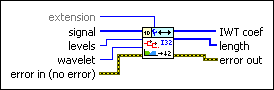extension specifies the method to use to pad data at the borders of the input signal. The extension length is equal to the length of the wavelet filters. When you select the extension method, make the transition between the input signal and the padded data as smooth as possible because a smooth transition generates fewer large detail coefficients and enhances the efficiency of the signal representation.

 0 Zero padding—Uses zeros to pad the input data. Watch for abrupt transitions between the padded zeros and the input data, which causes large artifacts near the transition. 1 Symmetric—Uses replications of the input data to pad the data, except that this VI left-flips the block at the input and right-flips the block at the end. 2 Periodic (default)—Adds a replication of the input data block before and another replication after the input data block to pad the data.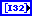signal specifies the input signal.levels specifies the number of levels in the discrete wavelet analysis. levels must be a positive integer no greater than log2(Ls), where Ls is the length of the 1D signal or the minimum dimensional size of the 2D signal. Otherwise, you can set the value to –1, which indicates that this VI sets levels as the largest integer no greater than log2(Ls). The default is –1.wavelet specifies the wavelet type to use for the integer wavelet analysis. The default is Haar. Options include Haar, bior2_2, and bior4_4 (FBI). If you use another type of wavelet, this VI uses the default value automatically.error in describes error conditions that occur before this node runs. This input provides standard error in functionality.IWT coef returns the approximation coefficients and the detail coefficients from the multi-level integer wavelet transform (IWT). This VI concatenates the coefficients into a 1D integer array starting with the approximation coefficients at the largest level followed by the detail coefficients at all levels in descending order. You can use the WA Get Coefficients of Integer Wavelet Transform VI to read the approximation coefficients or detail coefficients at a specific level. Wire IWT coef to the IWT coef input of the WA Get Coefficients of Integer Wavelet Transform VI.length returns, in a 1D array, the number of approximation and detail coefficients at each level. At a decomposition level of N, length is equal to N+2. The first element of length always is equal to the number of approximation coefficients. The last element of length indicates the total number of raw data samples. The length of the detail coefficients is arranged in descending order. You can use the WA Get Coefficients of Integer Wavelet Transform VI to read the approximation coefficients or detail coefficients at a specific level. Wire length to the length input of the WA Get Coefficients of Integer Wavelet Transform VI.error out contains error information. This output provides standard error out functionality.

## WA 2D Integer Wavelet Transform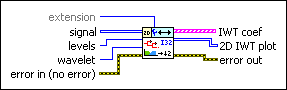extension specifies the method to use to pad data at the borders of the input signal. The extension length is equal to the length of the wavelet filters. When you select the extension method, make the transition between the input signal and the padded data as smooth as possible because a smooth transition generates fewer large detail coefficients and enhances the efficiency of the signal representation.

 0 Zero padding—Uses zeros to pad the input data. Watch for abrupt transitions between the padded zeros and the input data, which causes large artifacts near the transition. 1 Symmetric—Uses replications of the input data to pad the data, except that this VI left-flips the block at the input and right-flips the block at the end. 2 Periodic (default)—Adds a replication of the input data block before and another replication after the input data block to pad the data.signal specifies the 2D input signal.levels specifies the number of levels in the discrete wavelet analysis. levels must be a positive integer no greater than log2(Ls), where Ls is the length of the 1D signal or the minimum dimensional size of the 2D signal. Otherwise, you can set the value to –1, which indicates that this VI sets levels as the largest integer no greater than log2(Ls). The default is –1.wavelet specifies the wavelet type to use for the integer wavelet analysis. The default is Haar. Options include Haar, bior2_2, and bior4_4 (FBI). If you use another type of wavelet, this VI uses the default value automatically.error in describes error conditions that occur before this node runs. This input provides standard error in functionality.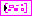IWT coef returns the approximation coefficients and the detail coefficients from the multi-level integer wavelet transform (IWT). Each element of the array includes the approximation coefficients and the detail coefficients at a level. Each element of the array contains the 2D IWT results of one level. The ith element stores the approximation coefficients and the detail coefficients at level i+1. You can use the WA Get Coefficients of Integer Wavelet Transform VI to read the approximation coefficients or detail coefficients at a specific level. Wire IWT coef to the IWT coef input of the WA Get Coefficients of Integer Wavelet Transform VI.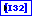low_low returns coefficients that are a low-resolution approximation of the original 2D signal.low_high returns the low_high coefficients. The high-frequency signal along the column direction influences the low_high coefficients.high_low returns the high_low coefficients. The high-frequency signal along the row direction influences the high_low coefficients.high_high returns the high_high coefficients. The high-frequency signal along the diagonal direction influences the high_high coefficients.2D IWT plot returns the approximation coefficients at the largest level and the detail coefficients at all levels. 2D IWT plot is the same size as signal.error out contains error information. This output provides standard error out functionality.

## WA Integer Wavelet Transform Details

Similarly to the discrete wavelet transform (DWT), this VI applies the IWT to the signal and then to the approximation coefficients of each level recursively.

On each level, this VI implements the IWT with the lifting scheme. The following illustration shows a flowchart of a one-level IWT with the lifting scheme: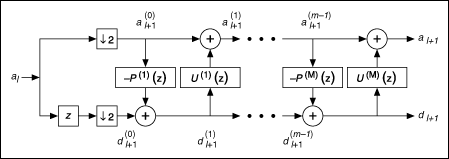al is the approximation coefficients of level l. al+1 and dl+1 are the approximation coefficients and the details coefficients of level l+1. The lifting scheme is an iterative procedure. The number of the iteration, M, depends on the wavelet type. At the first iteration, al splits into a set of even-indexed elements and a set of odd-indexed elements. This VI uses the set of even-indexed elements of the approximation coefficients of level l, al, 2k, as the initial approximation coefficients of level l+1,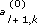. This VI uses the set of odd-indexed elements of the approximation coefficients of level l, al, 2k+1, as the initial detail coefficients of level l+1,. This VI then iteratively passes the initial approximation coefficients and the initial detail coefficients through the lifting filter, P(m)(z), and the dual lifting filter, U(m)(z), to obtain the approximation coefficients and the detail coefficients as shown in the following equations: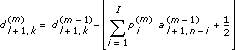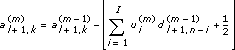where m indicates the index of iteration, k is the coefficient index, n is the total number of coefficients, I is the number of taps of the lifting filters, and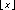is the operator to truncate x to the largest integer that is no greater than x. This VI computes M, P(m)(z), and U(m)(z) automatically according to the wavelet type you select.

The inverse IWT is a reverse operation of the IWT. The following illustration shows a flowchart of a one-level inverse IWT: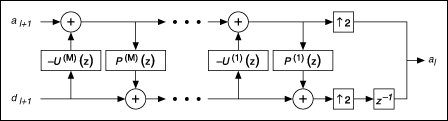Because IWT coefficients are integers, you do not need to quantize the coefficients in the process of compression, and you do not encounter the quantization error. Therefore, the IWT and the inverse IWT are used widely in lossless signal or image compression applications.

## References

• Daubechies, I., and W. Sweldens. "Factoring wavelet transforms into lifting steps." Technical Report, Bell Laboratories, Lucent Technologies (1996).
• Calderbank, R., I. Daubechies, W. Sweldens, and B. Yeo. "Wavelet transforms that map integers to integer." Applied and Computational Harmonic Analysis 5, no. 3 (1998): 332-369.

## Examples

Refer to the following VIs for examples of using the WA Integer Wavelet Transform VI:

• Lossless Medical Image Compression VI: labview\examples\Wavelet Analysis\WAApplications
• Integer Wavelet Transform on Image VI: labview\examples\Wavelet Analysis\WAGettingStarted
• Integer Wavelet Transform on 1D Signal VI: labview\examples\Wavelet Analysis\WAGettingStarted